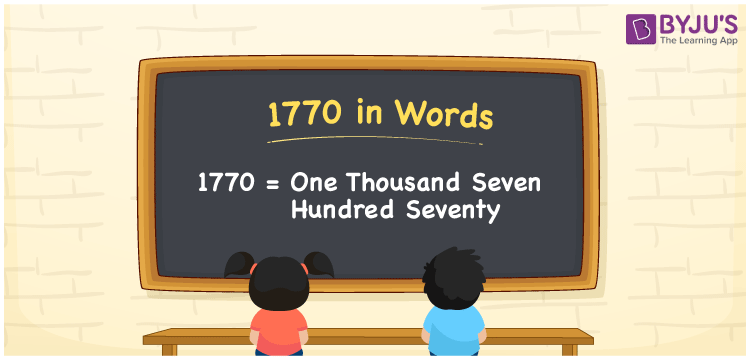# 1770 in Words

1770 in words is written as “One thousand seven hundred seventy”. In Maths, 1770 is a cardinal number that expresses a quantity. If the price of a table is Rs.1770, then it can be represented as Rupees One thousand seven hundred seventy, in English. Learn more about Numbers In Words at BYJU’S.

 1770 in Words One thousand seven hundred seventy One thousand seven hundred seventy in Numbers 1770

## 1770 in English Words## How to Write 1770 in Words?

The number 1770 in words can be written using a place value system, where we can identify the position of each digit. Since 1770 is a four-digit number, thus the place value of the digits is given by:

 Thousands Hundreds Tens Ones 1 7 7 0

From the above table,

1 → Thousands place

7 → Hundreds place

7 → Tens

0 → Ones place

Hence, when we read the number from right to left, it is One thousand seven hundred seventy.

### Expanded Form of 1770

We can write the expanded form as:

1 x Thousand + 7 × Hundred + 0 × Ten + 0 × One

= 1 x 1000 + 7 × 100 + 7 × 10 + 0 × 1

= 1000 + 700 + 70 + 0

= One thousand seven hundred seventy

1770 is a whole number that is succeeded by 1769 and preceded by 1771. Learn more about the number 1770 below:

• 1770 in Words – One thousand seven hundred seventy
• Is 1770 an odd number? – No
• Is 1770 an even number? – Yes
• Is 1770 a perfect square number? – No
• Is 1770 a perfect cube number? – No
• Is 1770 a prime number? – No
• Is 1770 a composite number? – Yes

## Frequently Asked Questions on 1770 in words

Q1

### What is 1770 in words?

1770 in words is given by One thousand seven hundred seventy.
Q2

### What is the rule to write 1770 in words?

1770 in words is written based on the place values of each digit. 1 is at thousands, 7 at hundreds, 0 at tens and 0 at ones. Thus, reading from right to left, it is One thousand seven hundred seventy.
Q3

### What is the value of 1770 + 30 in words?

1770 + 30 = 1800, i.e., One thousand eight hundred in words